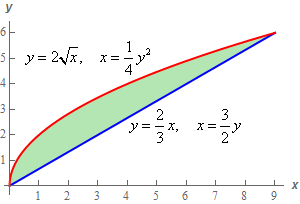Paul's Online Notes
Home / Calculus III / Multiple Integrals / Double Integrals over General Regions
Show Mobile Notice Show All Notes Hide All Notes
Mobile Notice
You appear to be on a device with a "narrow" screen width (i.e. you are probably on a mobile phone). Due to the nature of the mathematics on this site it is best views in landscape mode. If your device is not in landscape mode many of the equations will run off the side of your device (should be able to scroll to see them) and some of the menu items will be cut off due to the narrow screen width.

### Section 15.3 : Double Integrals over General Regions

2. Evaluate $$\displaystyle \iint\limits_{D}{{2y{x^2} + 9{y^3}\,dA}}$$ where $$D$$ is the region bounded by $$\displaystyle y = \frac{2}{3}x$$ and $$y = 2\sqrt x$$.

Show All Steps Hide All Steps

Start Solution

Below is a quick sketch of the region $$D$$.Note that we gave both forms of the equation for each curve to help with the next step.

In general, this sketch is often important to setting the integral up correctly. We’ll need to determine the order of integration and often the region will “force” a particular order. Many regions can only be dealt with easily by doing one particular order of integration and sometimes the only way to really see that is to have a sketch of $$D$$.

Even if you can do the integral in either order the sketch of $$D$$ will often help with setting up the limits for the integrals.

Show Step 2

Now, with this problem, the region will allow either order of integration without any real change of difficulty in the integration.

So, here are the limits for each order of integration that we could use.

$\begin{array}{*{20}{c}}\begin{array}{c}0 \le x \le 9\\ \displaystyle \frac{2}{3}x \le y \le 2\sqrt x \end{array}&{\hspace{0.25in}{\mbox{OR}}\hspace{0.25in}}&\begin{array}{c}0 \le y \le 6\\ \displaystyle \frac{1}{4}{y^2} \le x \le \frac{3}{2}y\end{array}\end{array}$ Show Step 3

As noted above either order could be done without much real change in difficulty. So, for this problem let’s integrate with respect to $$y$$ first.

Often roots in limits can lead to messier integrands for the second integration. However, in this case notice that both the $$y$$ terms will integrate to terms with even exponents and that will eliminate the root upon evaluation. This order will also keep the exponents slightly smaller which may help a little with the second integration.

Here is the integral set up for $$y$$ integration first.

$\iint\limits_{D}{{2y{x^2} + 9{y^3}\,dA}} = \int_{0}^{9}{{\int_{{\frac{2}{3}x}}^{{2\sqrt x }}{{2y{x^2} + 9{y^3}\,dy}}\,dx}}$ Show Step 4

Here is the $$y$$ integration.

\begin{align*}\iint\limits_{D}{{2y{x^2} + 9{y^3}\,dA}} & = \int_{0}^{9}{{\int_{{\frac{2}{3}x}}^{{2\sqrt x }}{{2y{x^2} + 9{y^3}\,dy}}\,dx}}\\ & = \int_{0}^{9}{{\left. {\left( {{y^2}{x^2} + \frac{9}{4}{y^4}} \right)} \right|_{\frac{2}{3}x}^{2\sqrt x }\,dx}}\\ & = \int_{0}^{9}{{4x\left( {{x^2}} \right) + \frac{9}{4}\left( {16{x^2}} \right) - \left[ {\frac{4}{9}{x^2}\left( {{x^2}} \right) + \frac{9}{4}\left( {\frac{{16}}{{81}}{x^4}} \right)} \right]\,dx}}\\ & = \int_{0}^{9}{{36{x^2} + 4{x^3} - \frac{8}{9}{x^4}\,dx}}\end{align*} Show Step 5

In this case there is a small amount of pretty simple simplification that we could do to reduce the complexity of the integrand and so we did that.

All that is left is to do the $$x$$ integration. Here is that work.

\begin{align*}\iint\limits_{D}{{2y{x^2} + 9{y^3}\,dA}} & = \int_{0}^{9}{{36{x^2} + 4{x^3} - \frac{8}{9}{x^4}\,dx}}\\ & = \left. {\left( {12{x^3} + {x^4} - \frac{8}{{45}}{x^5}} \right)} \right|_0^9 = \require{bbox} \bbox[2pt,border:1px solid black]{{\frac{{24057}}{5} = 4811.4}}\end{align*}